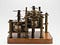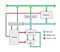# Calcutron-33: A Decimal Based RISC Microprocessor

## An imaginary CPU with a decimal number system instead of a binary number system to make teaching and learning how a CPU works easier.Part of the Analytical Engine, a mechanical computer which used decimal numbers rather than binary numbers for calculations just like the Calcutron-33.The Calcutron-33 Decimal RISC CPU Architecture. Registers contain 9 slots. Memory 90 slots. ALU adds and subtracts numbers. Decoder activates other boxes, by looking at content in Instruction Register.

## Example Progams

`    INP x2  // first number to multiply. This will get added    INP x3  // second number. Treated as counter    CLR x1  // accumulator for result. Clear it out.    nextdigit:    RSH x4, x3, 1  // Push right most digit of x3 into x4    multiply:    ADD x1, x1, x2 // Add first input to accumulator         DEC x4         // Decrement counter for number of additions    BGT x4, multiply  // Repeat while x4 > 0        LSH x2, x2, 1     // Left shift. x2 made 10x larger    BGT x3, nextdigit // check if all digits have been processed        OUT x1`

Written by